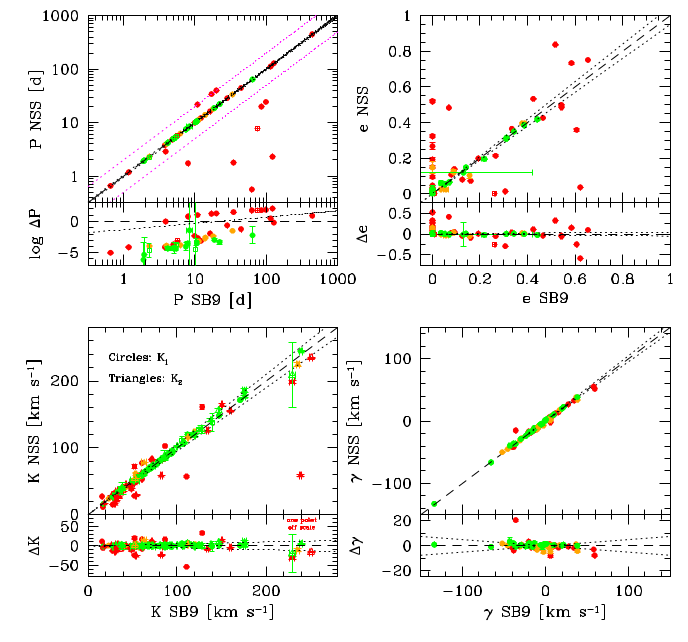# 7.5.5 Quality assessment and validation

Among the Gaia DR3 validation catalogues discussed in Section 7.4.6, only the SB9 and its subset restricted to Griffin’s data include SB2 solutions. The latter is not considered further because of the paucity of cross matches (only 12). For the whole SB9, there are 91 SB2 and 14 SB2C systems in common (out of a total of 647). In what follows the circular and eccentric solutions are discussed together.

Contrary to the SB1 case, the size of the deviations relative to the uncertainties is not used as a measure of the level of agreement with the reference parameters. It is because the large values often encountered make a graphical representation, as done in Section 7.4.6, inconvenient. The deviations are therefore discussed below in absolute terms. It is conceivable that the large differences can be attributed at least in part to the uncertainties that are generally underestimated, but this tentative statement deserves further investigation.Figure 7.50: Comparison for SB2 solutions with the SB9 parameters. Eccentric and circular solutions are shown as filled circles and open squares, respectively. The data are colour coded according to the deviations in σ units (see Section 7.4.6): below 3 (green), between 3 and 5 (orange), and above 5 (red). The uncertainties are often smaller than the size of the symbols. The long-dashed line in the upper panels is the 1:1 relationship. The dotted lines in all panels delineate an agreement at the 5% level. The short-dashed, magenta lines in the upper, left panel show the 1:2 and 2:1 relationships.

Figure 7.50 shows the comparison with the SB9 parameters. A total of 11 out of the 52 periods found ($\sim$21%) are not recovered to within 5%. For three of those, the NSS period is twice the SB9 one. There are no other orbital elements listed in the SB9 for these three binaries, but the RV curve folded with the literature period appears coherent. For the eccentricity, there are two main categories of outliers. First, very eccentric systems ($e$ $>$ 0.4) for which a larger dispersion is observed. This is somewhat expected and might be related to a different phase sampling of the RV curve in the two datasets. The second category is made up of systems that are flagged as (nearly) circular in one of the two catalogues, but not in the other. Several of them do not have a correct NSS period. For the RV semi-amplitude, excluding the obvious outlier, one obtains on average: $\langle\Delta K\rangle=-0.34\pm 7.62$ $\rm\,km\,s^{-1}$ (140 measurements). Excluding the clear outlier for the centre-of-mass velocity leads to $\langle\Delta\gamma\rangle=-0.42\pm 2.07$ $\rm\,km\,s^{-1}$ (70 measurements).Figure 7.51: Comparison between the mass ratios, q. Eccentric and circular solutions are shown as filled circles and open squares, respectively. The data are colour coded according to the deviations in σ units (see Section 7.4.6): below 3 (green), between 3 and 5 (orange), and above 5 (red). The uncertainties are often smaller than the size of the symbols. The long-dashed line in the upper panel is the 1:1 relationship. The dotted lines delineate an agreement at the 5% level.

Figure 7.51 shows the comparison between the mass ratios: $q$ = ${\cal{M}}_{2}$/${\cal{M}}_{1}$ = $K_{1}$/$K_{2}$. A total of 71 systems are depicted. Two regimes can be broadly identified, although the boundaries are quite uncertain given the relatively small number of systems. First, binaries with dissimilar masses ($q$ $<$ 0.75) for which Gaia DR3 seems to overestimate the mass ratio. Second, nearly equal mass systems with a much more satisfactory agreement, usually below the 5% level.